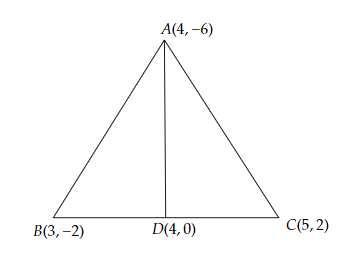# You have studied in Class IX, that a median of a triangle divides it into two triangles of equal areas. Verify this result for $├ó╦åŌĆĀABC$ whose vertices are $A (4, -6), B (3, -2)$ and $C (5, 2)$.

Given:

Vertices of a triangle are $A (4, -6), B (3, -2)$ and $C (5, 2)$.

To do:

We have to prove that the median of a triangle divides it into two triangles of equal areas.

Solution:

Let $A (4, -6), B (3, -2)$ and $C (5, 2)$ be the vertices of a triangle $ABC$.Let $D$ be the mid-point of the side $BC$ of $\triangle ABC$.

This implies,

Coordinates of point $D =$ Midpoint of BC $= (\frac{3+5}{2}, \frac{-2+2}{2})$

$=(\frac{8}{2}, 0)$

$=(4, 0)$

$AD$ is the median of $\triangle ABC$.

Area of $\triangle \mathrm{ABD}=\frac{1}{2}[x_{1}(y_{2}-y_{3})+x_{2}(y_{3}-y_{1})+x_{3}(y_{1}-y_{2})]$

$=\frac{1}{2}[4 \times(-2-0)+3(0+6)+4(-6+2)]$

$=\frac{1}{2}[-8+18-16]$

$=\frac{-6}{2}$

$=3$ sq units

Area of $\triangle \mathrm{ADC}=\frac{1}{2}[4(0-2)+4(2+6)+5\times(-6-0)]$

$=\frac{1}{2}[-8+32-30]$

$=\frac{-6}{2}$

$=3$ sq units

Area of $\triangle \mathrm{ABD}=$Area of $\triangle \mathrm{ADC}$

Therefore, the median of a triangle divides it into two triangles of equal areas.

Hence proved.